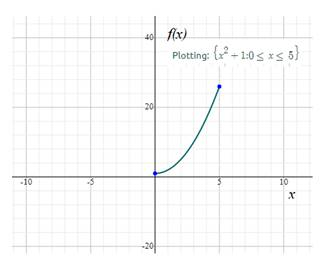# Find the domain of the function f ( x ) = x 2 + 1 , on the interval 0 ≤ x ≤ 5### Precalculus: Mathematics for Calcu...

6th Edition
Stewart + 5 others
Publisher: Cengage Learning
ISBN: 9780840068071### Precalculus: Mathematics for Calcu...

6th Edition
Stewart + 5 others
Publisher: Cengage Learning
ISBN: 9780840068071

#### Solutions

Chapter 2.1, Problem 46E
To determine

## Find the domain of the function f(x)=x2+1,  on the interval  0≤x≤5

Expert Solution

The domain of the function f(x)=x2+1 is [0,5]

### Explanation of Solution

Given information: Consider the function f(x)=x2+1

Calculation:

Recall the domain of a function is the set of input or argument values for which the function is real and defined.

Assume a function f(x)=x2+1 from the interval 0x5 values as shown in the figure below:From the above graph, the x values are limited to the values from x=0 to x=5

Thus, the domain of the function f(x)=x2+1 is [0,5]

### Have a homework question?

Subscribe to bartleby learn! Ask subject matter experts 30 homework questions each month. Plus, you’ll have access to millions of step-by-step textbook answers!